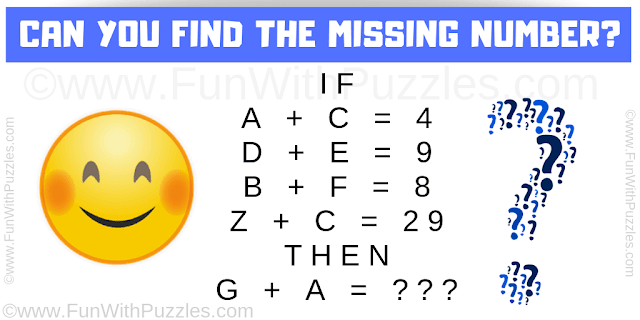This math logical reasoning question will tickle your mind. This is an easy math logic puzzle for kids. In this puzzle question, there are some equations in which some letters relate to the numbers. Your challenge is to decode this logical or mathematical relationship in these equations and find the value of the missing number which replaces the question mark.Can you solve this Math Logical Reasoning Question?

Answer of this "Math Logical Reasoning Question", can be viewed by clicking on the answer button.